• Citation:  ES Energy & Environment, 2020, 7, 48-55

DOI:10.30919/esee8c365

Received: 18 Nov 2019
Revised: 10 Dec 2019
Accepted: 11 Dec 2019
Published online: 15 Dec 2019

# High-Order Exceptional Points in Diffusive Systems: Robust APT Symmetry Against Perturbation and Phase Oscillation at APT Symmetry Breaking

Peichao Cao,1 Ying Li,2* Yugui Peng,1 Chengwei Qiu 2 and Xuefeng Zhu1*

1School of Physics and Innovation Institute, Huazhong University of Science and Technology, Wuhan, 430074, China

2Department of Electrical and Computer Engineering, National University of Singapore, Singapore 117583, Singapore

* Corresponding Author E-mail: [email protected]; [email protected]

## Abstract

In non-Hermitian systems with the Hamiltonians obeying parity-time (PT) symmetry, exploring the counterintuitive physics induced by degeneracies known as exceptional points (EPs) provides unprecedented ways to control energy flow. Recently, there are growing interests in bridging wave systems and diffusive systems, where anti-parity-time (APT) symmetry is demonstrated in diffusive systems. In this work, we start from the thermal energy transfer in a four-channel coupling model with the background flow velocities in adjacent channels opposite. A third order EP exists in this system, where temperature profiles in the moving channels are static in the APT symmetric phase (flow velocities below a threshold VEP at the EP), and the profiles begin to dynamically evolve in the APT broken phase (>VEP). By introducing a velocity perturbation into the background flow at the third order EP (VEP±∆v), we find APT symmetry keeps robust with the phases of temperature profiles in adjacent channels relatively static or locked. When ∆v is increased above a threshold (another EP), the APT symmetry is breaking with a transition from phase locking to phase oscillation, regardless of initial conditions. This work unveils rich physics in convectively coupled diffusive systems and offers us new prospects for the control of complex thermal fields.

## Table of ContentWe present high-order exceptional points in diffusive systems with robust APT symmetry against perturbation and phase oscillation at symmetry breaking.

Keywords: Anti-parity-time symmetry; Exceptional point; Phase transition; Heat transfer.

## 1. Introduction

Parity-time (PT) symmetry is attractive in quantum mechanics, since it allows for real eigenvalues in the non-Hermitian Hamiltonians that are associated with observable quantities in physical systems.1 For a physical system, the parity operator P and time reversal operator T acts based on the rules of Pψ(x)=ψ(-x) and Tψ(x)=ψ*(x), respectively, where *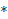denotes the complex conjugate.2 However, in PT symmetric systems, real eigenvalue is a conditional but not necessary result, since there exist phase transition points at which the PT symmetry will be spontaneously breaking and the eigenvalues become complex. Such phase transition points are termed as exceptional points (EPs), where eigenmodes are degenerate with both eigenvalues and eigenvectors coalesced.3,4 Here, EPs are completely different from diabolic points in the parameter spaces of Hermitian systems at which the eigenvectors are orthogonal.5,6 In the past two decades, the paradigm of PT symmetry in quantum mechanics has been successfully shifted into classical systems.7-13 For example, in optics and acoustics, the PT symmetry is constructed by introducing anti-symmetrically distributed gain and loss materials. New perspectives are envisioned in the PT symmetric platform, where various counterintuitive effects are theorectically proposed and experimentally demonstrated, such as unidirectional transparency,7,8 one-way cloaking,9,10 mode switching,11,12 EP sensing,13,14 coherent lasing and absorption.15,16

Recently, it was found that EPs also exist in anti-parity-time (APT) symmetric systems, at which phase transition occurs with the eigenvalues changing from pure imaginary (APT symmetric phase) into complex (APT broken phase).17,18 Unlike the PT symmetric Hamiltonian that satisfies PTH=HPT, the APT symmetric Hamiltonian follows the rule of PTH=-HPT.19,20 Mathematically, the PT symmetric Hamiltonian can be transformed into the APT symmetric one by simply multiplying the Hamiltonian with an imaginary number i. Physically, for a tight-binding model, this operation will end up with a pure virtual coupling between two tight-binding sites, which is quite challenging for the practical implementation. In optics, the virtual coupling between adjacent meta-atoms is realized in an indirect and complicated way by adding a well-designed third meta-atom in-between to equivalently generate a virtual coupling action.19,21 It needs to be mentioned that the diffusive systems (e.g., thermal systems) are inherently non-Hermitian. The most interesting is the notion that the coupling in diffusive systems is originally imaginary. Thus, with the aid of convection and low diffusivity, we can imitate various wave-like dynamics in the framework of diffusive systems.22 For example, the stable temperature profile under low diffusivity, which mimics a wave packet, can stop or even move in the opposite direction against the background flow via convection couplings, corresponding to zero or negative group velocity of a wave packet.

In this work, we explore the phase transition in a four-channel coupling thermal system, which, compared with the two-channel toy model,22 provides a higher degree of freedom to control energy flow. When the background flow velocities in neighboring channels are opposite, there exists a third order EP in the eigenspectrum, accompanied with APT symmetry breaking. Adding a perturbation into the background flow velocity at the high order EP (vEP±∆v), the APT symmetry remains robust, where the phases of temperature profiles in adjacent moving channels are relatively static, as manifested in a locked mode. When the perturbation ∆v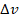surpasses a threshold (another EP), the whole system will transit into the broken APT symmetry. In this case, we observe the effect of robust phase oscillation that is irrelevant to the initial conditions, induced by the concealing dimension in the high order EP. Just as the transformation thermotics that rapidly developed together with the transformation optics and acoustics,23-26 APT phase transition at EPs in diffusive systems, inspired from the PT phase transition in classical wave systems, will promisingly expand the vision of counterintuitive thermal flow regulation.

2. Model and theory

Figs.1 (a&b) show the schematics of 3D model and the corresponding 2D model, respectively. The four identical ring-shaped channels are stacked along z-axis, where the thickness, inner radius and outer radius of each channel are b, R1 and R2, respectively. Adjacent channels are coupled through an oil layer with the thickness d. Rotation velocities of the background flow in the four channels are set to be Ω1,3=v/R1 and Ω2,4=-v/R1, respectively, given that R1≈R2.Fig. 1 (a), (b) The schematics of 3D model and the corresponding 2D model. Here, the channel width is b. The thickness of oil layers is d. The inner radius of the ring-shaped channel is R1. The outer radius is R2. The velocities of background flows in adjacent channels are Ω=±v/R1. (c) The imaginary part and real part of eigenvalues vs. the background flow velocity. Red dots at A and B mark the EPs.

Diffusive systems are dissipative with energy exchanges to the environment, which can be regarded as inherently non-Hermitian systems. In stark contrast with wave systems that are governed by the real and Hermitian Hamiltonians, diffusive systems are featured with pure imaginary Hamiltonians. Recently, great efforts have been made to marry the two different scenarios, where the introduced background flow velocity in the convection process can serve as the effective group velocity for directional energy flows, on condition that the thermal diffusivity of materials is trivial. In this case, the Hamiltonians of diffusion systems become complex, with the APT symmetry possibly constructed and EPs generated. For the model displayed in Fig. 1, the convection-diffusion equations describing the temperature field evolutions take the forms ofwhere T1,2,3,4 are the temperature profiles in channels 1, 2, 3, 4. ρr and Cr are the mass density and heat capacity of the ring materials, respectively. In Eq. (1), D=κrρrCr is the thermal diffusivity of the ring materials, with κr denoting the thermal conductivity. For the coupling oil layers, hs1,s2,s3,s4 and κo represent the coupling strengths between adjacent channels and the thermal conductivity of oil, respectively.

Eq.(1) is based on the continuity of temperature fields on the boundaries of oil layers. For simplicity, we first consider the thermal flow in a slowly rotating ring with trivial diffusivity D→0. The convection-diffusion equation is expressed into. Since the diffusion term is a perturbation compared to the advection term, the convection-diffusion equation can be reduced into a homogeneous form, which has a wave form solution T(x,t)=Aei(kx-ωt). Substituting the wave form solution back into the inhomogeneous convection-diffusion equation, we will end up with ω=-ik2D+kv. In poor thermal conducting materials, the initial temperature field profile keeps unchanged during the rotation. After one circle, the field coincides, where we define the perimeter L=2πR1 as the periodic wavelength of the circulating energy packets. Straightforwardly, the wave number k in the wave form solution can be defined as k=2π/L=1/R1. Eq.  (1) actually takes a similar form to the time-independent Schrödinger equation Hψ(x)=Eψ(x), where the Hamiltonian is(2)

with S0=-i(k2D+h) and h=κoρrCrbd. After some derivations, eigenvalues of the Hamiltonian in Eq. (2) are solved by(3)

In Eq. (3), the imaginary part of eigenvalues is mainly determined by the coupling strength h, which characterizes the decay rate of thermal energy. The eigenvalues will turn into complex ones, on condition that the effect of convection outweighs the coupling action. As shown by Eq. (3), ω±1 and ω±2 are complex with real and imaginary parts, when h < kh and $\sqrt{2}h, respectively. Eigenvectors of the Hamiltonian corresponding to ω±1 and ω±2 are derived to beFigure 1(c) shows the relation between the eigenvalues and the flow velocity. In the calculation, we set R1=0.1 m, R2=0.11 m, d=5 mm, b=1 mm, κr=100 W/(m·K), κo=1 W/(m·K), ρro=1000 kg/m3 and Cr=Co=1000 J/(kg·K). Thus we have k=1/R1=10 m-1 and       D=κr / ρrcr=10-4m2/s.  In Fig. 1(c), there exist two EPs in the spectrum, as marked by the red dots A and B. From Eqs. (3) and (4), we obtain that the degenerated point A at v=hk is a third order EP with the eigenvalues being ω±1,-2=-i(k2D+h) and ω+2=-i(k2D+3h). The corresponding eigenvectors are u±1,-2=(-1,i,i,1)T and u+2=(-1,2+i,-2+i,1)T, respectively. The degenerated point B at $v=\sqrt{2}h/k$ is a typical two order EP with the eigenvalues ω±1=-i(k2D+h)±h and ω±2=-i(k2D+2h). The eigenvectors are and . Here, it should be mentioned that the observed field evolution in diffusive systems eventually follows the minimum loss route, where the high-loss eigenfields will damp rapidly. As a result, in Fig. 1(c), the whole system would take the branch of ω-1, as marked by the brown lines. Therefore, as the flow velocity increases, the system will only experience the third order EP at vEP=h/k=2 cm/s, where the APT breaking phase transition occurs. Here we emphasized that the originally degenerated real parts of eigenvalues split into the upper and lower branches after the EPs, where we take the lower branch as ω-1,-2 for consistency, as shown in Fig. 1(c) and the following.

## 3. Results and discussion

From the Eq.(3), for vEP, all eigenvalues are pure imaginary. Substituting ϕ=arcsin(v/vEP) into Eq.(4),the eigenvectors can be further simplified intoCombining the forward and backward wave form solutions, we will obtain expressions of eigenstates T=[T1,T2,T3,T4]=e-iωt[u(k)eikx+u(-k)e-ikx]. where T1,2,3,4 denote the steady temperature profiles in channels 1-4. All the possible eigenstates with eigenfields in the four channels are derived as followsNote that the whole system ends up with the minimum loss case of T-1 over time, showing that the steady-state temperature fields will stand still and phase differences between T1(T4) and T2(T3) are ±ϕ=±arcsin(v/vEP). This claim is demonstrated from the full wave simulation results by using a finite element solver COMSOL Multiphysics® 5.3, as shown in Fig. 2(a), where the initial temperature fields in all channels are set by T1,2,3,4=293.15+100y (K). At v=vEP, namely, the third order transition point of eigenstates from APT symmetry to APT symmetry breaking, the temperature fields in each channel keep standing still and the phase difference between adjacent channels is π/2. To be specific, Fig. 2(b) shows the eigenfields distribution T1,2,3,4 in the four eigenstates T±1,-2~-2[coskx, sinkx, sinkx, -coskx] and T+2~-2[coskx,-$\sqrt{5}$cos(kx+θ-π/2), $\sqrt{5}$cos(kx-θ-π/2), -coskx] (θ=arctan1/2).

For v>vEP, the whole system transits into the APT breaking phase, where the evolution of temperature profiles becomes very complicated. Intuitively, the convection effect outperforms thermal coupling, making the system impossible to reach a steady state. Substituting ψ=arccosh(v/vEP) into Eq. (4) , we will derive the eigenstates asIn inspection of Eq. (7), the amplitudes of forward and backward wave form components in the eigenstate T-1 are A-1,1=-e, B-1,1=-e-2ψ, A-1,2=eψ, B-1,2=e, A-1,3=eψ, B-1,3=e and A-1,4=B-1,4=1. Obviously, the temperature fields T1,2,3,4 are unstable over time evolution. Here we utilize the local maximum to trace the position (or phase) of temperature fields in each channel. From ∂Tj/∂x=0 and Eq. (7), we will obtain the relation tankxj=tan[Re(ω)t](A-1,j-B-1,j)/(A-1,j+B-1,j). Define the phase difference between channels 1 and 2 as φ1-φ2=k(x1-x2). Considering

tank(x1-x2)=$\frac{\mathrm{tan}k{x}_{1}-\mathrm{tan}k{x}_{2}}{1+\mathrm{tan}k{x}_{1}×\mathrm{tan}k{x}_{2}}$，we haveFig. 2 APT symmetry breaking at the high order EP in the four-channel diffusive system. (a) The time evolution of phase differences φ1-φ2 and φ4-φ3 vs. the flow velocity v, where we set )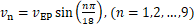. (b) The eigenstates T±1 and T±2 at the EP. In (a) and (b), T1,2,3,4 and φ1,2,3,4 denote the steady temperature profiles and the related phases in channels 1-4. (c), (d) Theory and simulation results of time evolution of φ1-φ2 at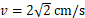, when the APT symmetry is broken.

3.2 Perturbation at the high order EP

In this section, we explore the case that a perturbation is introduced to the high order EP. Here we introduce a flow velocity modulation ±∆v into the four-channel system, as schematically shown in Fig. 3(a). In this case, the Hamiltonian containing the perturbation is rewritten as follows（9）

The imaginary part and the real part of the Hamiltonian are shown in Figs. 3(b) and 3(c). The result shows that as the modulation strength ∆v increases from 0cms, there exist three EPs. One is the third order EP A' at ∆v=0cms, while the typical two order EPs B' and C' locate at ∆v=0.57cms and ∆v=1.6cms. As we mentioned before, the diffusive system is always following the lowest dissipation state over time. Therefore, the eigenvalue of the Hamiltonian takes the brown branch in Figs. 3(b) and 3(c). The results show that the APT symmetry is robust against the weak perturbation at the high order EP. When the perturbation strength is above the threshold ∆v=1.6cms, the whole system will transit into APT symmetry breaking across the EP C' and the temperature fields become unstable. Figure 3(d) displays the time evolution of phase difference φ1-φ2 at different modulation strengths, where the APT is symmetric. In Fig. 3(d), we find that the phases of steady-state temperature profiles in adjacent circulating channels are relatively static (t > 60 s), as manifested in a locked mode with a nearly constant phase difference. To be specific, the phase differences of three locked modes (in degrees) are 55° for ∆v=0.5cms, 41.2° for ∆v=1cms, and 25.6° for ∆v=1.5cms, respectively.Fig. 3 Perturbation at the high order EP. (a) The schematic of flow velocity modulation in the four channels. (b), (c) The imaginary part and real part of eigenvalues vs. the flow velocity modulation ∆v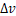, with the brown lines denoting the scenario of the lowest energy dissipation. Red dots at A', B', and C' mark the EPs. (d) The phase difference φ1-φ2 between channels 1 and 2 over time evolution in APT symmetry, where ∆v=0.5 cm/s, 1.0 cm/s, 1.5 cm/s.

As aforementioned, the temperature fields in diffusive systems are unstable in the APT broken phase. Here we show that by introducing flow velocity modulation at the high order EP, the phase difference between adjacent channels will oscillate over time instead of continuously diverging at APT breaking. In addition, the phase oscillation at APT breaking is independent with the initial condition. In Figs. 4(a)-4(c), we present a numerical demonstration of the phase oscillation effect at APT breaking with three different initial conditions. Specifically, in Fig. 4(a), the temperature profile at t=0 s is T1,3=293.15+100x (K) and T2,4=293.15+100y (K), with φ1-φ2=-90°. In Fig. 4(b), the temperature profile at t=0 s is T1,2,3,4=293.15+100y (K), with φ1-φ2=0°. In Fig. 4(c), the temperature profile at t=0 s is 293.15-100x (K) and T2,4=293.15+100y (K), with φ1-φ2=90°. Here the flow velocity modulation strength ∆v=4 cm/s. The results reveal that for the three cases, phase oscillation occurs at t>40 s, with the oscillation center angle φcenter=45° and the time cycle Tperiod=32 s. In Figs. 4(a) - 4(c), the insets show the temperature profiles in channels 1 and 2 at different times when the phase difference φ1-φ2 takes maximum or minimum values, vividly displaying the relative periodic oscillation of phase difference over time. Figure 4(d) shows that both the oscillation center angle and the time cycle decrease as the flow velocity modulation strength increases. When the flow modulation strength ∆v is much larger than the EP velocity vEP, for example ∆v=40 cm/s, the flows in channels 1 and 2 can be regarded as almost synchronous circulation. Therefore, the phase oscillation is weak with the center angle close to 0 (φcenter=1.4°), while the time cycle of the phase jittering Tperiod=21.6 s.Fig. 4 Periodic phase oscillation in APT symmetry breaking. (a), (b) and (c) Phase oscillation of φ1-φ2 at ∆v=4 cm/s with the initial condition being φ1-φ2=-π/2, 0 and π/2 at t=0 s, respectively. (d) The oscillation center angles/time cycles vs. the flow velocity modulation ∆v.

At last, we would like to briefly discuss the cases of more channels coupled, odd-number channels coupled and the suggestions for experiment. When more channels are coupled, we can basically obtain four-order EPs and beyond. In this case, the technical difficulty for experimental observation is increasing. Higher ordered EPs are intuitively associated with very complicated behaviors in phase evolution. However, the properties protected by APT symmetry are expected to be unchanged. For example, the phase differences of temperature profiles between adjacent channels for the steady states are locked in the APT symmetric case. Phase oscillation at APT symmetry breaking is also supposed to occur when the higher ordered EP is perturbed with the velocity modulation.

In this work, we focus on the model of even-number coupled channels with inside flows having equal-but-opposite velocities. It will be interesting if an additional channel is introduced to break the symmetry of the whole system (i.e., odd-number channels). In light of the Hamiltonian analysis, there will exist an isolated branch with no conjugate pair in the eigenvalue spectrum, which does not degenerate with other paired branches. When the eigenmodes on the isolated branch have the minimum loss, the system will follow this single branch and do not experience EP-induced phase transition. For the experimental demonstration, we can use nylon rings (